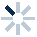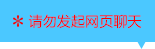| | |

||
APP端下载AndroidiPhone
|
￥72

|
2080人点赞
124186人已学习
|

3天无理由退款

3天无理由退款：退款将以超级币形式退至您的超级课堂学习账户，便于您重新选购其他课程。恶意退款将被冻结账号。

• 1、复合函数的定义，要弄清楚外层函数，内层函数，直接变量，中间变量，因变量这些概念
2、 我们看待复合函数的方式，只是为了更好的研究它，其中中间变量是拆卸和组装复合函数的关键
3、 注意“内层函数的函数值，是外层函数的自变量”，所以内层函数的值域与外层函数的定义域的交集必须非空
• 1、讲解几道复合函数的题目，在做题时，可以用“换元法”的视角来求复合函数的解析式，将内层函数整体代入
• 1、学习两种基本的图象变换——关于$y$轴的对称变换和$x$轴方向上的平移变换
2、 前者很简单，只要把$x$变成$-x$就$OK$，后者要注意“孤立$x{}$"，看它的变化，遵循左加右减的规律
• 1、在研究两个函数间图象的对称与平移变换关系时，要专注地看自变量$x$的变化
2、 推出一个一般结论的图象平移公式，$\dfrac{b_{2}-b_{1}}{k}$
• 1、综合关于$y$轴的对称变换和$x$轴方向上的平移变换，可以采用两步走策略。“先对称，再平移”或“先平移，再对称”，两种方式异曲同工
• 1、注意括号同级原理，$f(a)$中的$a$和$f[g(a)]$中的$a$根本不是同一个$a$
2、 根据括号同级原理，前者$a$相当于后者的$g(a)$$f[g(a)]$的求法
• 1、如果已知复合函数的解析式，就直接带入
2、 如果给出外层和内层函数$f(x)$与$g(x)$各自的解析式。你就有两种选择：1、把复合函数组装起来，再代入。2、先代入内层函数求值，再代入外层函数求值
3、 反过来，我们也能通过$f[g(a)]$的值，求$a$值，或者求未知参数
• 1、$f(a)$的两种基本求法：（1）解$x$代入法：通过$g(x)=a$解出$x$的值，再代入复合函数的解析式；（2）整体代入法：通过代数变形，把复合函数用内层函数的整体表示，直接带入求值
• 1、掌握“括号范围相同”这个重要的结论
2、 在这个结论的指导下，我们就能求出复合函数的定义域，实现定义域的自由切换
• 1、学习求复合函数定义域的三种题型：已知$f(x)$的定义域，求$f[g(x)]$的定义域。已知$f[g(x)]$的定义域，求$f(x)$的定义域。已知$f[g(x)]$的定义域，求$f[h(x)]$的定义域
2、 只要牢牢抓住“括号范围相同”这个法则，就能在各种函数的定义域之间切换自由
• 1、已知解析式，由内向外，逐层求值域
2、 对于二次函数和反比例函数，最好配合图像分析值域
3、 如果人为规定了定义域，也是按这个顺序算
4、 反过来，规定了值域，也能由外向内求解集
• 1、若解析式未知，对于$f[g(x)]$与$f(x)$，若括号范围相同，即$g(x)$的值域和$x$的定义域相同，则$f[g(x)]$与$f(x)$的值域相同
2、 若前者不同，后者的值域是不确定的，可能相同，也可能不同
• 1、形如$f(x)=\frac{p(x)}{q(x)}$叫做分式函数，其中$p(x)$、$q(x)$是既约整式且$q(x)$的次数不低于一次
2、 其中形如$f(x)=\frac{(ax+b)}{(cx+d)}$的是一次分式函数。注意$c\neq 0$，且$ad\neq bc$
3、 分子为常数的一次分式函数，可由反比例函数的图像横向平移得到
• 1、对于分子含有自变量的一次分式函数，要用分离常数法把解析式恒等变形为常数分离的形式，再考虑横向和纵向的平移
2、 分离常数法的经典步骤，第一步：分子分母系数化1；第二步：在分子中制造约分项；注意恒等变形；第三步：约分，分离常数
3、 函数平移后对称中心的确定，注意由于“左加右减”，对称中心的横坐标要加负号。而当解析式不是常数分离的形式时，要用分离常数法处理，才能快速准确地找到对称中心
• 1、通过三道例题，向同学们展示了分离常数法和函数平移方法在题目中的具体应用
• 1、对于一般的一次分式函数，用分离常数法处理后，求出其对称中心，在反比例函数定义域和值域的基础上，用横坐标代替$0$，写出定义域；以纵坐标代替$0$，写出值域
2、 对于人为规定了定义域的一次分式函数，有两种方法求值域。一种是通过平移，画出一次分式函数的图像，然后在图像上，直接根据定义域找值域，另一种是分层求值域，这种方法避免了平移画图的复杂性
• 1、求解一类特殊的高次分式函数值域时，用换元法处理成一次的，就完全没有任何难度了
• 1、由复合函数$f[g(x)]$和内层函数$g(x)$的解析式，求$f(x)$解析式这类常见题型，主要有两种解法：换元法，令$t$等于内层函数，再把$x$也转化$t$的式子，最后换元，代入复合函数解析式，求出$f(t)$，即$f(x)$，注意不要忘记求出t的取值范围
2、 配凑法，将复合函数的解析式凑配出含有内层函数结构的形式，从而直接将$g(x)$换为$x$，得到$f(x)$的解析式
• 1、换元法和配凑法在题目中的应用，其实就是括号同级原理、换元等量运算和代数式恒等变形，这三块内容的综合运用，同一个对应关系$f$，不停地变换着外形罢了
• 1、​当题目告诉你函数类型时，就可以使用待定系数法，设出解析式，然后直接代入求系数
• 1、介绍了如何利用特殊函数值代入和恒等思想求解待定系数
2、 到这里，你又掌握了求函数解析式的第二样神器——待定系数法，在已知函数类型的题目中，就可以用它大显身手
• 1、对于第一种赋值法——特殊值赋值法，如果给了你特殊值，就用此方法
• 1、对于第二种赋值法——内部赋值法，如果内部的两个函数，内部赋值后恰好位置调换，就能用此法
• 复合函数解析式求法综合练习

• 1梦！
• 2超级学员1231607
• 3超级学员1464258
• 4CHJF
• 5超级课堂
• 6超级学员2790848
• 7人___
• 8超级学员3627998
• 9马冰雨
• 10WJGN194328人在学
￥ 78 ￥ 78125003人在学
￥ 78 ￥ 78217320人在学
￥ 0 ￥ 0124186人在学
￥ 72 ￥ 72

• 0
• 点击分享有好礼
••app端下载关注微信号